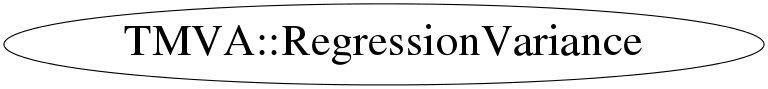# class TMVA::RegressionVariance

## Function Members (Methods)

public:
 virtual ~RegressionVariance() static TClass* Class() TString GetName() Double_t GetSeparationGain(const Double_t& nLeft, const Double_t& targetLeft, const Double_t& target2Left, const Double_t& nTot, const Double_t& targetTot, const Double_t& target2Tot) virtual Double_t GetSeparationIndex(const Double_t& n, const Double_t& target, const Double_t& target2) virtual TClass* IsA() const TMVA::RegressionVariance& operator=(const TMVA::RegressionVariance&) TMVA::RegressionVariance RegressionVariance() TMVA::RegressionVariance RegressionVariance(const TMVA::RegressionVariance& s) virtual void ShowMembers(TMemberInspector& insp) const virtual void Streamer(TBuffer&) void StreamerNVirtual(TBuffer& ClassDef_StreamerNVirtual_b)

## Data Members

protected:
 TString fName name of the concrete Separation Index impementation

## Class Charts## Function documentation

Double_t GetSeparationGain(const Double_t& nLeft, const Double_t& targetLeft, const Double_t& target2Left, const Double_t& nTot, const Double_t& targetTot, const Double_t& target2Tot)
``` Separation Gain:
the measure of how the quality of separation of the sample increases
by splitting the sample e.g. into a "left-node" and a "right-node"
(N * Index_parent) - (N_left * Index_left) - (N_right * Index_right)
this is then the quality crition which is optimized for when trying
to increase the information in the system
for the Regression: as the "Gain is maximised", the RMS (sqrt(variance))
which is used as a "separation" index should be as small as possible.
the "figure of merit" here has to be -(rms left+rms-right) or 1/rms...
```
Double_t GetSeparationIndex(const Double_t& n, const Double_t& target, const Double_t& target2)
``` Separation Index:  a simple Variance
```

```default constructor
```
`{fName = "Variance for Regression";}`
RegressionVariance(const TMVA::RegressionVariance& s)
```copy constructor
```
`{}`
virtual ~RegressionVariance()
``` destructor
```
`{}`

``` Return the name of the concrete Index implementation
```
`{ return fName; }`